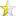### C++``123456789101112131415161718192021222324252627282930313233`` ``````#include #include #include /** * \brief Apply the ROT13 algorithm to a string. * \param source Source text to apply the algorithm to. * \return The transformed text is returned. */ std::string ROT13(std::string source) { std::string transformed; for (size_t i = 0; i < source.size(); ++i) { if (isalpha(source[i])) { if ((tolower(source[i]) - 'a') < 14) transformed.append(1, source[i] + 13); else transformed.append(1, source[i] - 13); } else { transformed.append(1, source[i]); } } return transformed; } int main() { std::string source; std::cout << "Enter the source text: " << std::flush; std::getline(std::cin, source); std::cout << "Result: " << ROT13(source) << std::endl; return 0; }``````